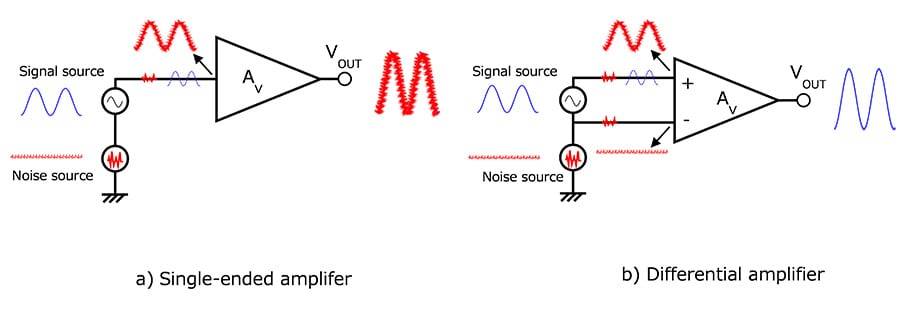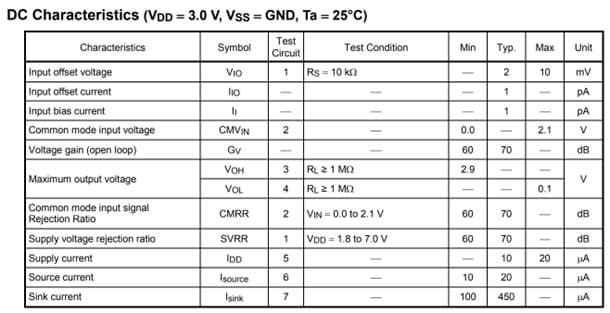# What is the purpose of using a differential amplifier such as an op amp? (Common-mode rejection ratio: CMRR)

Differential amplifiers are used mainly to suppress noise.

Noise consists of typical noise (differential noise) and common-mode noise. Since the differential amplifier amplifies the difference between the two inputs (Non-inverting input VIN(+) and inverting input VIN(-)) , it can easily attenuate common-mode noise in which the same noise is applied to the differential input terminals.

There are two main causes of common-mode noise:
1.Noise is generated in the wires and cables, due to electromagnetic induction, etc., and it causes a difference in potential (i.e., noise) between the signal source ground and the circuit ground.
2.Current flowing into the ground of a circuit from another circuit causes a ground potential rise (noise).

VOUT = AV x [{VIN(+) + Vnoise} - {VIN(-) + Vnoise}]
= AV x {VIN(+) - VIN(-)}

In both 1 and 2 above, the ground potential, a reference for a circuit, fluctuates because of noise. It is difficult to remove common-mode noise with typical filters. Differential amplifiers are used as a means of suppressing common-mode noise.
The op-amp configures this differential amplifier as the main circuit. The symbol in Fig. 1 shown below represents a differential amplifier (operational amplifier) . It has two inputs: VIN(+) and VIN(-). The output voltage is equal to a difference in voltage between the two inputs multiplied by the amp’s gain (AV):

VOUT = AV x {VIN(+) - VIN(-)}

In this way, common-mode noise superimposed on the op amp input stage is eliminated. However, if noise is superimposed on the GND or power supply of the op amp, this noise will be superimposed on the output.Fig. 2 Common mode noise superimposed on the input of the amplifier

Suppose that common-mode noise (Vnoise) is superimposed on the differential inputs in Fig. 2 (b) . Then,

VIN(+)‘ = VIN(+) + Vnoise

VIN(-)‘ = VIN(-) + Vnoise

Hence, the output is expressed as follows. This indicates that the differential amplifier cancels out common-mode noise:

VOUT = AV x [ { VIN(+) + Vnoise } - { VIN(-) + Vnoise } ] = AV x { VIN(+) - VIN(-) }

The common-mode rejection ratio (CMRR) is specified as one of the electrical characteristics of an op-amp.（See Table-1 Example of electrical characteristics in the data sheet )

CMRR is the ratio of common mode gain to differential gain.
Theoretically, the op amp should not amplify the common mode signal at all. However, in reality, due to the effects of internal element variations, etc., there is a slight effect of a common-mode signal on the output terminal. For this reason, CMRR is standardized as a measure for removing common-mode noise.
This factor is a minute deviation of the input voltage due to the input offset voltage.
(Please refer to FAQ: What is the input offset voltage of an op-amp?)

What is the input offset voltage of an op-amp?

The offset voltage is specified on the datasheet with input voltage = VDD/2. However, this voltage is not a fixed value and varies depending on the magnitude of the input voltage.

For this reason, the circuit shown in Fig. 3 is defined by the following formula.
CMRR = 20 x log ( | ( VIN1 – VIN2) / ( VOUT1 – VOUT2 ) | x ( RF + RS ) / RS )
When VIN = 0.0 V, VIN = VIN1 and VOUT = VOUT1
When VIN = 2.5 V, VIN = VIN2 and VOUT = VOUT2Table-1 Example of an electrical characteristics table in a datasheet (TC75S55FU)